6.4.5 Aside: Initial Black Insertion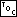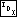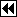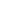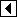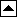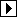The traditional algorithm for insertion in an RB tree colors new nodes red. This is a good choice, because it often means that no rebalancing is necessary, but it is not the only possible choice. This section implements an alternate algorithm for insertion into an RB tree that colors new nodes black.

The outline is the same as for initial-red insertion. We change the newly inserted node from red to black and replace the rebalancing algorithm:

```210. <RB item insertion function, initial black 210> =
void **rb_probe (struct rb_table *tree, void *item) {
<rb_probe() local variables 198>

<Step 1: Search RB tree for insertion point 199>
<Step 2: Insert RB node; RB_RED => RB_BLACK 200>
<Step 3: Rebalance after initial-black RB insertion 211>

return &n->rb_data;
}
```

The remaining task is to devise the rebalancing algorithm. Rebalancing is always necessary, unless the tree was empty before insertion, because insertion of a black node into a nonempty tree always violates rule 2. Thus, our invariant is that we have a rule 2 violation to fix.

More specifically, the invariant, as implemented, is that at the top of each trip through the loop, stack pa[] contains the chain of ancestors of a node that is the black root of a subtree whose black-height is 1 more than it should be. We give that node the name q. There is one easy rebalancing special case: if node q has a black parent, we can just recolor q as red, and we're done. Here's the loop:

```211. <Step 3: Rebalance after initial-black RB insertion 211> =
while (k >= 2)   {
struct rb_node *q = pa[k - 1]->rb_link[da[k - 1]];

if (pa[k - 1]->rb_color == RB_BLACK)       {
q->rb_color = RB_RED;
break;
}

if (da[k - 2] == 0)
{         <Left-side rebalancing after initial-black RB insertion 212>       }
else       {         <Right-side rebalancing after initial-black RB insertion 216>       }
}
```

This code is included in 210.

Consider rebalancing where insertion was on the left side of q's grandparent. We know that q is black and its parent pa[k - 1] is red. Then, we can divide rebalancing into three cases, described below in detail. (For additional insight, compare these cases to the corresponding cases for initial-red insertion.)

```212. <Left-side rebalancing after initial-black RB insertion 212> =
struct rb_node *y = pa[k - 2]->rb_link;

if (y != NULL && y->rb_color == RB_RED)
{     <Case 1 in left-side initial-black RB insertion rebalancing 213>   }
else   {
struct rb_node *x;

if (da[k - 1] == 0)
y = pa[k - 1];
else       {         <Case 3 in left-side initial-black RB insertion rebalancing 215>       }

<Case 2 in left-side initial-black RB insertion rebalancing 214>
}
```

This code is included in 211.

Case 1: q's uncle is red

If q has an red “uncle” y, then we recolor q red and pa[k - 1] and y black. This fixes the immediate problem, making the black-height of q equal to its sibling's, but increases the black-height of pa[k - 2], so we must repeat the rebalancing process farther up the tree: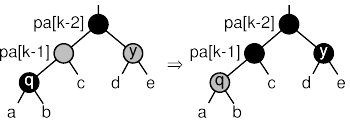```213. <Case 1 in left-side initial-black RB insertion rebalancing 213> =
pa[k - 1]->rb_color = y->rb_color = RB_BLACK;
q->rb_color = RB_RED;
k -= 2;
```

This code is included in 212 and 217.

Case 2: q is the left child of pa[k - 1]

If q is a left child, then call q's parent y and its grandparent x, rotate right at x, and recolor q, y, and x. The effect is that the black-heights of all three subtrees is the same as before q was inserted, so we're done, and break out of the loop.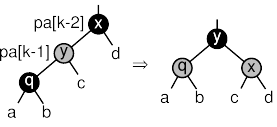```214. <Case 2 in left-side initial-black RB insertion rebalancing 214> =
x = pa[k - 2];
x->rb_color = q->rb_color = RB_RED;
y->rb_color = RB_BLACK;

pa[k - 3]->rb_link[da[k - 3]] = y;
break;
```

This code is included in 212.

Case 3: q is the right child of pa[k - 1]

If q is a right child, then we rotate left at its parent, which we here call x. The result is in the form for application of case 2, so after the rotation, we relabel the nodes to be consistent with that case.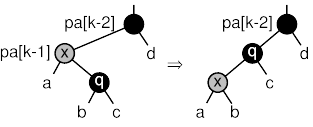```215. <Case 3 in left-side initial-black RB insertion rebalancing 215> =
x = pa[k - 1];
y = pa[k - 2]->rb_link = q;
```

This code is included in 212.

6.4.5.1 Symmetric Case

```216. <Right-side rebalancing after initial-black RB insertion 216> =
struct rb_node *y = pa[k - 2]->rb_link;

if (y != NULL && y->rb_color == RB_RED)
{     <Case 1 in right-side initial-black RB insertion rebalancing 217>   }
else   {
struct rb_node *x;

if (da[k - 1] == 1)
y = pa[k - 1];
else       {         <Case 3 in right-side initial-black RB insertion rebalancing 219>       }

<Case 2 in right-side initial-black RB insertion rebalancing 218>
}
```

This code is included in 211.

```217. <Case 1 in right-side initial-black RB insertion rebalancing 217> =
<Case 1 in left-side initial-black RB insertion rebalancing 213>
```

This code is included in 216.

```218. <Case 2 in right-side initial-black RB insertion rebalancing 218> =
x = pa[k - 2];
x->rb_color = q->rb_color = RB_RED;
y->rb_color = RB_BLACK;

pa[k - 3]->rb_link[da[k - 3]] = y;
break;
```

This code is included in 216.

```219. <Case 3 in right-side initial-black RB insertion rebalancing 219> =
x = pa[k - 1];
y = pa[k - 2]->rb_link = q;